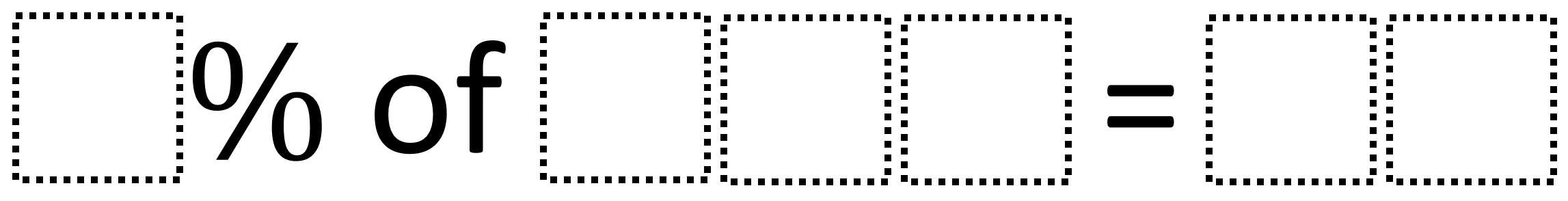Home > Grade 6 > Percentages

# Percentages

Directions: Using the digits 0 to 9 at most one time each, fill in the boxes to create a correct sentence:### Hint

How can we figure out a way to make the two-digit number not have a decimal?

### Answer

There are many answers including:5% of 320=16
5% of 260=13
5% of 620=31
2% of 650=13
6% of 350=21

Source: Cecilia Calvo

## Subtracting Multi-Decimals

Directions: Use the digits 1 to 9, at most one time each, to fill in …

### 5 comments

1.Nice! I was going to submit one in a similar vein.

Using the numbers 1-9, at most one time each, fill in the boxes to create a correct sentence:

[] [] % of [] [] is [] []?

68% of 25 is 17
25 % 68 of is 17

25% of 76 is 19
76% of 25 is 19

92% of 75 is 69…

All of the solutions involve a 75 or a 25

Significantly harder:
Three digits!

Using the numbers 0-9, at most one time each, fill in the boxes to create a correct sentence:

[] [] [] % of [] [] []is [] [] [] ?

375 % 184 is 690.

348% of 175 is 609.

2.One answer out of many possible answers is 5% of 260 is 13

3.4.My student got 8% of 950= 76. Is this a possible highest answer?